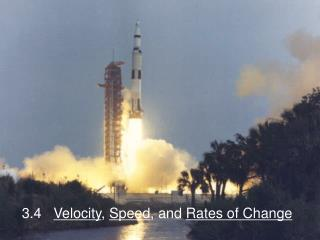# 3.4 Velocity , Speed , and Rates of Change - PowerPoint PPT PresentationDownload Presentation3.4 Velocity , Speed , and Rates of Change

3.4 Velocity , Speed , and Rates of ChangeDownload Presentation## 3.4 Velocity , Speed , and Rates of Change

- - - - - - - - - - - - - - - - - - - - - - - - - - - E N D - - - - - - - - - - - - - - - - - - - - - - - - - - -
##### Presentation Transcript

1. 3.4 Velocity, Speed, and Rates of Change

2. Change in position is measured in both displacement and distance The difference between the starting and ending positions of an object The total amount of “ground covered” by an object or the total length of its path If you were to drive 10 miles east and then 4 miles west 10 miles east 4 miles west Displacement = 6 miles Distance = 14 miles

3. position time And the position graph would look like this: 10 miles east 4 miles west Displacement = 6 miles Distance = 14 miles

4. B position (miles) A time (hours) (The velocity at one moment in time.) Consider a graph of position (distance from a starting point) vs. time. Average velocity can be found by taking: The speedometer in your car does not measure average velocity, but instantaneous velocity.

5. Velocity is the first derivative of position. Mr. Murphy’s fall from the 196 foot platform can be expressed with the equation: …where t is in seconds and s is measured in feet. Given the above statement, the equation for his velocity is: …which is expressed in what units? feet/second

6. Find Which is easy enough except…32 what? t (seconds) First, let’s look at the graph of velocity. Note that like the position graph, s(t), the y-axis represents velocity while the x axis represents time v(t) (feet/second)

7. Since the slope of a line is based on: …or in this case: …we are now talking about: Acceleration Is an expression of… Acceleration which is a rate of change of velocity. feet/second2

8. Remember: If you ever get lost, what can always save you? Acceleration is the derivative of velocity. UNITS! feet/second2

9. Gravitational Constants: Speed is the absolute value of velocity. Example: Free Fall Equation

10. Speed is the absolute value of velocity. Wait! So what is the difference between speed and velocity? If the object is moving upward… …or to the right If the object is moving downward… …or to the left But speed does not indicate direction so speed will always be positive…

11. Speed is the absolute value of velocity. Wait! So what is the difference between speed and velocity? If an object is moving upward at a speed of 40 ft/sec… If an object is moving downward at a speed of 40 ft/sec But regardless of the direction, the speed will always be positive…

12. position time It is important to understand the relationship between a position graph, velocity and acceleration: WAIT! How can you tell that acceleration is positive? The slope (velocity) is tilting upward so the slope (velocity) is increasing acc neg vel pos & decreasing acc neg vel neg & decreasing acc zero vel neg & constant acc zero vel pos & constant acc pos vel neg & increasing velocity zero acc pos vel pos & increasing acc zero, velocity zero

13. position time Based on this graph, how can you use velocity and acceleration to determine when an object is speeding up or slowing down? acc neg vel pos & decreasing acc neg vel neg & decreasing acc zero vel neg & constant acc zero vel pos & constant acc pos vel neg & increasing velocity zero acc pos vel pos & increasing acc zero, velocity zero time

14. position time Slowing Down Speeding up acc neg vel pos & decreasing acc neg vel neg & decreasing acc zero vel neg & constant acc zero vel pos & constant acc pos vel neg & increasing velocity zero acc pos vel pos & increasing acc zero, velocity zero time

15. Slowing Down Speeding up v and a have the same sign v and a have opposite signs So the moral of the story is…

16. Average rate of change = Instantaneous rate of change = Rates of Change: These definitions are true for any function. ( x does not have to represent time. ) p